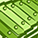# QlikView App Dev

Discussion Board for collaboration related to QlikView App Development.

Announcements
Join us for a live Q&A! September 21, 10 AM ET - Onboarding Fast in Qlik Sense SaaS! REGISTER
cancel
Showing results for
Did you mean:Champion

## Save All Field Names In New TableAll,

I want a script that should store all the field names alone in the document. For example, If I have 5 tables and 35 fields in a document, I want to store all the fields names in a new table or qvd.

1 Solution

Accepted SolutionsMaster III

Hi This is the script from

let vTabName = 'DataStructures';

let vLoadStart = num(now() - today(), '#.########', '.', ',');

for i = 0 to NoOfTables() - 1

for ii = 1 to NoOfFields(TableName(\$(i)))

let vTableName = '[' & TableName(\$(i)) & ']';

let vFieldName = '[' & FieldName(\$(ii), TableName(\$(i))) & ']';

let vFieldCounter = 0;

for j = 0 to NoOfTables() - 1

for jj = 1 to NoOfFields(TableName(\$(j)))

let vFieldNameCounter = '[' & FieldName(\$(jj), TableName(\$(j))) & ']';

let vFieldCounter = if('\$(vFieldName)' = '\$(vFieldNameCounter)', 1 + \$(vFieldCounter), \$(vFieldCounter));

next

next

DataStructure:

\$(i) as TableNumber,

TableName(\$(i)) as TableName,

NoOfRows(TableName(\$(i))) as Rows,

\$(ii) as FieldNumber,

FieldName(\$(ii), TableName(\$(i))) as FieldName,

Count(\$(vFieldName)) as FieldTotalCount,

Count(distinct \$(vFieldName)) as FieldDistinctCount,

MissingCount(\$(vFieldName)) as FieldMissingCount,

TextCount(\$(vFieldName)) as FieldTextCount,

NumericCount(\$(vFieldName)) as FieldNumericCount,

NullCount(\$(vFieldName)) as FieldNullCount,

num(Count(\$(vFieldName)) / NoOfRows(TableName(\$(i))), '#.##0%') as FieldDensity,

if(TextCount(\$(vFieldName)) >= 1 and NumericCount(\$(vFieldName)) >= 1, 'mixed',

if(TextCount(\$(vFieldName)) >= 1, 'string', 'numeric')) as FieldFormat,

if(\$(vFieldCounter) > 1, 'yes (' & \$(vFieldCounter) & ')', 'no') as Key

Resident \$(vTableName) Where '\$(vTableName)' <> 'DataStructure';

let vFieldCounter = 0;

next

next

store DataStructure into \$(pApp@03)DataStructure.qvd (qvd);

HTH

6 RepliesPartner

Hello,

Check Fieldname() and TableName() from Help.

BR

SerhanChampion
Author

Hi Serhan,

I have lots of tables in my document and wants to store all them in a new table. So I guess the loop should be correct solution for the above scenario. As I am new to qlikview, struggling to get the desired result.

Thanks

TamilPartner

Hello,

Right. Loop would solve.

Something like

for i = 0 to nooffields('tablename')

next i

You can even try it with a nested loop (loop for tables too). There is nooftables() function as well. You can send the table name in the above example parametric with tablenumber().

Just trying pseudo because I don't have the exact code but it's more than possible with the technique above.

BR

SerhanMaster III

FOR i = 0 to NoOfTables() - 1

LET vTableName = TableName(\$(i));

LET vOutfile = '\$(vTableName)' & '_' & '\$(vPeriodo).csv';

STORE [\$(vTableName)] INTO [\$(vOutfile)] (ansi, txt, delimiter is ';');

NEXTMaster III

Hi This is the script from

let vTabName = 'DataStructures';

let vLoadStart = num(now() - today(), '#.########', '.', ',');

for i = 0 to NoOfTables() - 1

for ii = 1 to NoOfFields(TableName(\$(i)))

let vTableName = '[' & TableName(\$(i)) & ']';

let vFieldName = '[' & FieldName(\$(ii), TableName(\$(i))) & ']';

let vFieldCounter = 0;

for j = 0 to NoOfTables() - 1

for jj = 1 to NoOfFields(TableName(\$(j)))

let vFieldNameCounter = '[' & FieldName(\$(jj), TableName(\$(j))) & ']';

let vFieldCounter = if('\$(vFieldName)' = '\$(vFieldNameCounter)', 1 + \$(vFieldCounter), \$(vFieldCounter));

next

next

DataStructure:

\$(i) as TableNumber,

TableName(\$(i)) as TableName,

NoOfRows(TableName(\$(i))) as Rows,

\$(ii) as FieldNumber,

FieldName(\$(ii), TableName(\$(i))) as FieldName,

Count(\$(vFieldName)) as FieldTotalCount,

Count(distinct \$(vFieldName)) as FieldDistinctCount,

MissingCount(\$(vFieldName)) as FieldMissingCount,

TextCount(\$(vFieldName)) as FieldTextCount,

NumericCount(\$(vFieldName)) as FieldNumericCount,

NullCount(\$(vFieldName)) as FieldNullCount,

num(Count(\$(vFieldName)) / NoOfRows(TableName(\$(i))), '#.##0%') as FieldDensity,

if(TextCount(\$(vFieldName)) >= 1 and NumericCount(\$(vFieldName)) >= 1, 'mixed',

if(TextCount(\$(vFieldName)) >= 1, 'string', 'numeric')) as FieldFormat,

if(\$(vFieldCounter) > 1, 'yes (' & \$(vFieldCounter) & ')', 'no') as Key

Resident \$(vTableName) Where '\$(vTableName)' <> 'DataStructure';

let vFieldCounter = 0;

next

next

store DataStructure into \$(pApp@03)DataStructure.qvd (qvd);Serhan, Sasidhar: Thanks a lot.to both of you.